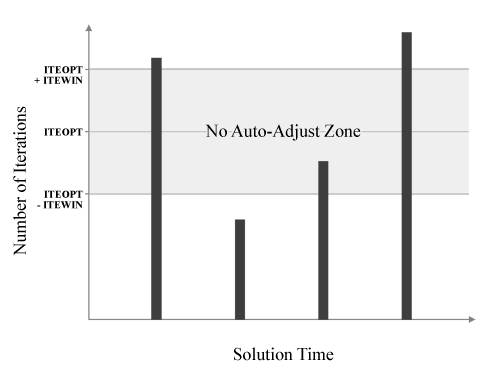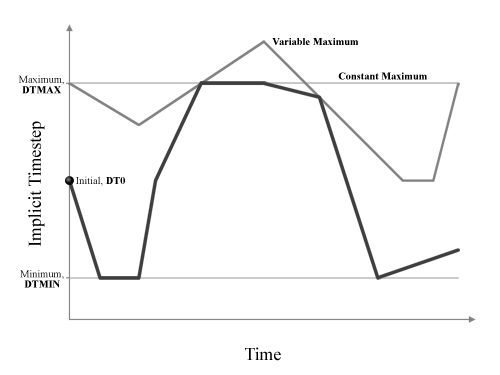Automatic Timestep Control for Implicit Simulations

The known advantage with implicit simulations is that the solution is unconditionally stable allowing larger values of timestep. In implicit static simulations (IMASS=0 in *CONTROL_IMPLICIT_DYNAMICS), the simulation time has no real significance but is rather an indication of the applied load magnitude. For example if we have a simulation where a load is applied linearly such that it reaches 100% of its magnitude at the termination time of 100seconds and the current simulation time is 20seconds using an arbitrary timestep of 1second, then we can interpret that our load (assuming linear scale) is 20% of its maximum load. In the case of implicit dynamic simulations (IMASS=1) time takes real meaning and unlike explicit solution, the timestep is unconditionally stable and thus allows us to choose a large timestep.

By default, LS-DYNA uses a constant implicit timestep, specified using DT0 in *CONTROL_IMPLICIT_GENERAL , for the entire duration of the simulation time. The magnitude of DT0 is usually choose as a fraction of the total simulation time and is entirely dependent on the nonlinearity of the problem. Using a constant timestep is very conservative in nature since irrespective of the number of iterations each timestep was required to converge, the timestep is unchanged and this may result in large number of expensive iterations. For a problem whose non-linearity is unknown, this is actually not a bad thing since smaller timesteps pose lesser nonlinearity issues and may be easier to converge. However, once the user becomes familiar with a problem, careful usage of automatic implicit timestep control in LS-DYNA is a very good alternative to reduce the number of iterations thereby reducing the simulation time.

Automatic timestep control is activated using *CONTROL_IMPLICIT_AUTO by setting AUTO = 1. The built-in logic in LS-DYNA then takes over to â€œauto-adjustâ€? the timestep and the logic is purely based on the number of iterations that was taken at the current timestep. The parameter ITEOPT (default is 11) allows the user to specify an â€œoptimumâ€? number of iterations which is to be used to decide where a auto-adjust the current timestep. If the number of iterations to converge at the current timestep is greater than ITEOPT, LS-DYNA then reduces the current timestep (using a built in scale-factor) in an attempt to reduce the non-linearity of the problem. However, if the number of iterations at the current timestep is less than ITEOPT, LS-DYNA then increases the current timestep (using a built-in scale-factor) assuming the problem is getting in easier to solve. To allow some tolerance to the value of ITEOPT, the parameter ITEWIN is a very useful feature which prevents LS-DYNA to hastily auto-adjust the timestep. When ITEWIN is defined (default is 15) and the number of iterations at the current timestep falls in the rage of ITEOPT +/- ITEWIN, then LS-DYNA bypasses the the auto-adjust of the timestep. This is shown in the following figure.To provide a minimum allowable timestep size that LS-DYNA can auto-adjust to, the parameter DTMIN can be specified. To defined maximum allowable timestep, there are two possible ways using the parameter DTMAX. When DTMAX is greater than zero, then LS-DYNA will not auto-adjust such that the timestep is either equal to or below DTMAX. When DTMAX is less than zero, it then refers to a load curve which can be used to define a variable maximum allowable timestep. This is illustrated in the following figure.A Few Important Notes on Using Automatic Timestep Control
As mentioned above, the auto timestepping is purely based on the number of iterations that was taken at the current timestep. It does not understand any issues related to contact-impact interactions. For cleaner contact interaction, a smaller timestep is preferable which when violated, results in contact breakdown or instability due to the application of large penalty forces. To aid better contact treatment while maximizing the advantages of auto-timestepping feature, the value of DTMAX must be carefully chosen such that large values do not cause contact difficulties. In the case of contact breakdown, LS-DYNA may still converge and yield a solution but with interpenetration which should be avoided. Unless a user becomes familiar with a problem, a constant timestep is a safer method and as the experience with the problem increases, a good auto time stepping can significantly reduce simulation time while maintaining the accuracy of the solution.

•Bin Zhang says:

Hi, Suri,

My name is Bin & I have a question about the Load Curve which is used in a Dyna Implicit Static model:

*DEFINE_CURVE
curve2
2 0 1.0 1.0 0.0 0.0 0
0.0 0.0
1.0 0.0
2.0 1.666667
3.0 1.666667
4.0 0.0
5.0 0.0

Thanks,

Bin Zhang

•Suri Bala says:

Bin,

The load will be based on the load curve. Hence it will be the value at time t=3s which is 1.666667.

Suri

•Bin Zhang says:

Hi, Suri,

Thank you for your quick response.

I have a curve for Gravity Load as follows:
*DEFINE_CURVE
curve1
1 0 1.0 1.0 0.0 0.0 0
0.0 9810.0
1.0 9810.0
3.0 9810.0
5.0 9810.0

If the load is based on the curve, during the whole process (0-5s) the gravity load is all the same (?). But I checked the results such as Deflection at some nodes, when t = 0, the deflection = 0, then goes up. How to explain this?

Thanks & best regards,

Bin

•Subutay says:

Hi,

i have a question about usage of automatic time step control. It’s very useful sometimes especially when convergence is a problem. However, when i use this option often i observe that steps of loading are missed. If there is a load rump up or any other change (e.g. decrease after rumping up), auto option misses to follow the load aplication curve. Is there a way to fix this?

Thanks,

•Suri Bala says:

Subutay,

Can you please review Figures 8.5 and 8.6. All you need to do is to define
“key points” and LS-DYNA will update the timestep to ensure equilibrium is
acheived at these “key points”.

Suri